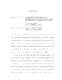## Image representation and compression via sparse solutions of systems of linear equations2012
##### Authors
Nava Tudela, Alfredo
We are interested in finding sparse solutions to systems of linear equations $mathbf{A}mathbf{x} = mathbf{b}$, where $mathbf{A}$ is underdetermined and fully-ranked. In this thesis we examine an implementation of the {em orthogonal matching pursuit} (OMP) algorithm, an algorithm to find sparse solutions to equations like the one described above, and present a logic for its validation and corresponding validation protocol results. The implementation presented in this work improves on the performance reported in previously published work that used software from SparseLab. We also use and test OMP in the study of the compression properties of $mathbf{A}$ in the context of image processing. We follow the common technique of image blocking used in the JPEG and JPEG 2000 standards. We make a small modification in the stopping criteria of OMP that results in better compression ratio vs image quality as measured by the structural similarity (SSIM) and mean structural similarity (MSSIM) indices which capture perceptual image quality. This results in slightly better compression than when using the more common peak signal to noise ratio (PSNR). We study various matrices whose column vectors come from the concatenation of waveforms based on the discrete cosine transform (DCT), and the Haar wavelet. We try multiple linearization algorithms and characterize their performance with respect to compression. An introduction and brief historical review on the topics of information theory, quantization and coding, and the theory of rate-distortion leads us to compute the distortion $D$ properties of the image compression and representation approach presented in this work. A choice for a lossless encoder $gamma$ is left open for future work in order to obtain the complete characterization of the rate-distortion properties of the quantization/coding scheme proposed here. However, the analysis of natural image statistics is identified as a good design guideline for the eventual choice of $gamma$. The lossless encoder $gamma$ is to be understood under the terms of a quantizer $(alpha, gamma, beta)$ as introduced by Gray and Neuhoff.